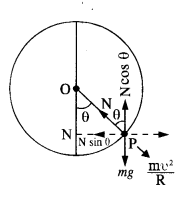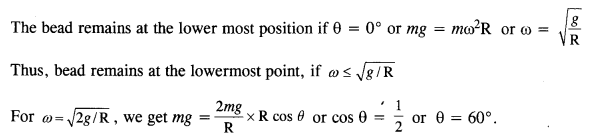## NCERT Solutions for Class 11 Physics Chapter 5 Laws of Motion

These Solutions are part of NCERT Solutions for Class 11 Physics. Here we have given NCERT Solutions for Class 11 Physics Chapter 5 Laws of Motion

Question 1.
Give the magnitude and direction of the net force acting on
(a) a drop of rain falling down with a constant speed.
(b) a cork of mass 10 g floating on water.
(c) a kite skilfully held stationary in the sky.
(d) a car moving with a constant velocity of 30 km/h on a rough road.
(e) a high-speed electron in space far from all gravitating objects, and free of electric and magnetic fields.
(a) (As the drop of rain is falling with constant speed, therefore, according to Newton’s first law of motion, the net force on the drop of rain is zero, orsince v = u = constant, therefore, F =0.
(b) As the cork is floating on water, its weight is balanced by the upthrust due to water. Hence net force on cork is zero.
(c) Since kite is held stationary, in accordance with first law of motion, the net force on the kite is zero.
(d) Since car is moving with a constant velocity, the net force on the car is zero.
(e) Since the high speed electron in space is far from all gravitating objects and free of electric and magnetic fields, the net force on electron is zero.

Question 2.
A pebble of mass 0.05 kg is thrown vertically upwards. Give the direction and magnitude of the net force on the pebble

1. during its upward motion,
2. during its downward motion,
3. at the highest point where it is momentarily at rest. Do your answers change if the pebble was thrown at an angle of say 45° with the horizontal direction? Ignore air resistance.

1. When the pebble is moving upward the force acting on it is a gravitational force in a downward direction.
F = mg = 0.05 × 10 = 0.5 N
2. Even in this case F = mg = 0.5 N in downward direction.
3. Since there is no force other than gravitational force acting on a pebble, during the whole process F = mg = 0.5 N. Note that the pebble moves in opposite direction because of its initial velocity. The situation remains the same for pebble thrown at an angle.

Question 3.
Give the magnitude and direction of the net force acting on a stone of mass 0.1 kg.
(a) just after it is dropped from the window of a stationary train,
(b) just after it is dropped from the window of a train running at a constant velocity of 36 km/h,
(c) just after it is dropped from the window of a train accelerating with I ms-2,
(d) lying on the floor of a train which is accelerating with 1 ms-2, the stone being at rest relative to the train. Neglect air resistance throughout.
(a) In this case F = weight of stone = Mg = 0.1 x 9.8 = 0.98 N, (vertically downwards)

(b)
Since no force acts on the stone due to the motion of the train,
∴ F = 0.98 N (vertically downwards)

(c)
The stone will experience an additional force F’ (along horizontal) ie.,
F’ = Ma = 0.1 x 1= 0.1 N
As the stone is dropped, the force F’ no longer acts and the net force acting on the stone is
F = Mg = 0.98 N (vertically downwards)

(d)
In this case, the weight of the stone is balanced by the normal reaction. The net force on the stone is given by F’ = Ma = 0.1 x 1 = 0.1 N (horizontally)

Question 4.
One end of a string of length is connected to a particle of mass m and the other to a small peg on a smooth horizontal table. If the particle moves in a circle with speed υ the net force on the particle (directed towards the center) is :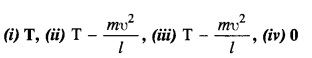T is the tension in the string. [Choose the correct alternative].
(i) The centripetal force necessary for the particle to move in a circular path is provided by the tension in the string. Hence net force on the particle is nothing but tension T in the string.

Question 5.
A constant retarding force of 50 N is applied to a body of mass 20 kg moving initially with a speed of 15 ms-1. How long does the body take to stop?
Here M = 20 kg, F = -50 N [retarding force]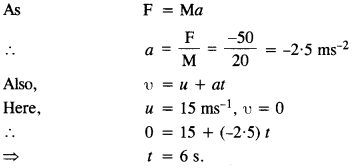Question 6.

A constant force acting on a body of mass 3.0 kg changes its speed from 2.0 ms-1 to 3.5 ms-1 in 25 s. The direction of the motion of the body remains unchanged. What is the magnitude and direction of the force?Mass of body, M = 3 kg
Force acting on the body, F = Ma = 3 x 0.06 = 0.18 N
Since the applied force increases the speed of the body; it acts in the direction of motion.

Question 7.
A body of mass 5 kg is acted upon by two perpendicular forces 8 N and 6 N. Give the magnitude and direction of the acceleration of the body.
Here, F1 = 8 N and F2 = 6 N
The magnitude of the resultant force acting on the body is given by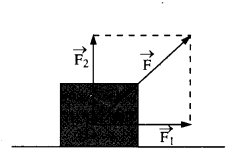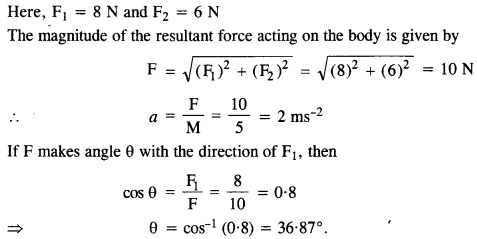Question 8.
The driver of a three-wheeler moving with a speed of 36 km/h sees a child standing in the middle of the road and brings his vehicle to rest in 4.0 s just in time to save the child. What is the average retarding force on the vehicle? The mass of the three-wheeler is 400 kg and the mass of the driver is 65 kg.0 = 10 + 4a                                                              .                ‘
=>a = – 2.5 m s-2
∴ Magnitude of retarding force = 465 x 2.5 = 1162.5 N

Question 9.
A rocket with a lift-off mass of 20,000 kg is blasted upwards with an initial acceleration of 5.0 ms-2. Calculate the initial thrust (force) of the blast.
Given m = 20000 kg
a = 5ms-2 (against gravity)
since the rocket has to move upwards against gravity the total initial thrust of the blast is given by
F = ma + mg
= m (a + g) = 20000 (5 + 9.8)
= 296 × 105 N.

Question 10.
A particle of mass 0-40 kg moving initially with a constant speed of 10 ms1 to the north is subject to a constant force of 8.0 N directed towards the south for 30 s. Take the instant the force is applied to be t = 0, the position of the particle at that time to be x = 0, and predict its position at f = -5s, 25 s, 100 s.
Here m = 0.40 kg, h = 10 ms1, F = – 8N (retarding force)Question 11.
A truck starts from rest and accelerates uniformly with 2.0 ms-2. At t = 10 s, a stone is dropped by a person standing on the top of the truck (6 m high from the ground). What are the
(a) velocity, and
(b) acceleration of the stone at t = 11s? (Neglect air resistance).
(a) Here, u = 0, a = 2.0 ms-2, t = 10 s, g = 9.8 m s-2
Velocity along horizontal direction, υx = u + at = 0 + 2 x 10 = 20 ms1
After Is (11 s – 10 s), velocity along vertical direction, υy =u + gt = 0 + 9.8 X 1 = 9.8 m s1
Therefore, resultant velocity,Question 12.
A bob of mass 0.1 kg hung from the ceiling of a room by a string 2 m long is set into oscillation. The speed of the bob at its mean position is 1 ms-1. What is the trajectory of the bob if the string is cut when the bob is
(1) at one of its extreme positions,
(2) at its mean position?
1. When the bob is at one of its extreme positions its velocity is zero. Hence if the string is cut, it will fall straight down due to gravitational force.

2. At the mean position the bob has a horizontal velocity of 1 m/s. If the string is cut, bob is acted by vertical gravitational force = a = 9.8 ms-2. Hence bob will behave like a projectile and follows a parabolic path.

Question 13.
A man of mass 70 kg stands on a weighing scale in a lift which is moving

1. upwards with a uniform speed of 10 ms-1,
2. downwards with a uniform acceleration of 5 ms-2,
3. upwards with a uniform acceleration of 5 ms-2, What would be the readings on the scale in each case?
4. What would be the reading if the lift mechanism failed and it hurtled down freely under gravity?

The weighing machine measures the reaction R which is nothing but the apparent weight.
1. when the lift is moving upwards with uniform speed.
R = mg = 70 × 9.8 = 686 N.

2. When lift moves downwards with an acceleration of 5m/s²
R = m (g – a) = 70 (9.8 – 5) = 336 N.

3. When lift moves upwards with with an acceleration of 5m/s²
R = m (g + a) = 70 (9.8 + 5) = 1036 N.

4. If the lift falls down freely under gravity
R = m (g – g) = 0.

Question 14.
Figure shows the position-time graph of a particle of mass 4 kg. What is the
(1) force on the particle for t < 0, t > 4 s, 0 < f < 4 s ?
(2) (impulse at t = 0 and t = 4 s ? (Consider one-dimensional motion only).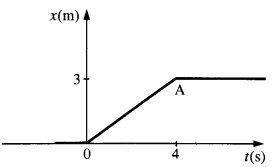1. From the position time graph we can see that the particle is in rest during t < 0 and t > 4. Hence net force on it is zero t < 0, t > 4 s. During 0 < t < 4; the graph has a constant slope i.e, particle
has uniform velocity = 3/4 = 0.75 m/s.
Hence net force is zero.

2. at t = 0 u = 0 v = 0.75
impulse = change in momentum
= M (v – u) = 4 (0.75 – 0)
= 3 kg m/s
at t = 4, u = 0.75 v = 0
impulse = 4 (0 – 0.75) = – 3 kg m/s.

Question 15.
A horizontal force of 600 N pulls two masses 10 kg and 20 kg (lying on a frictionless table) connected by a light string. What is the tension in the string ? Does the answer depend on which mass end the pull is applied ?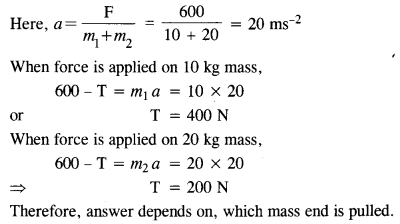Question 16.
Two masses 8 kg and 12 kg are connected at the two ends, of a light in extensible string that goes over a frictionless pulley. Find the acceleration of the masses and the tension in the string when the masses are released.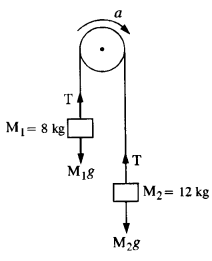Let M1 = 8 kg and M2 = 12 kg
Let T be the tension in the string and the acceleration with which the system moves is a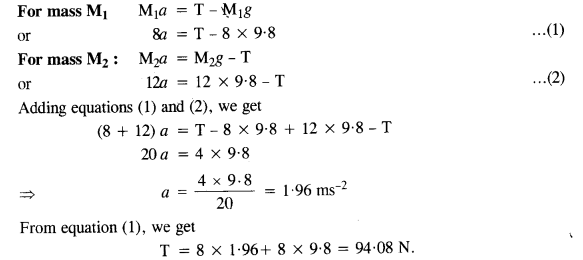Question 17.
A nucleus is at rest in the laboratory frame of reference. Show that if it disintegrates into two smaller nuclei the products must be emitted in opposite directions.
Let M = Initial mass of the nucleus
Mand M2 are masses after disintegration and υ1 and υare their respective velocities.
Now, initial momentum of the nucleus = M x 0 = 0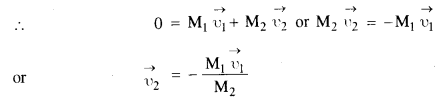Here, a negative sign shows that the products will be emitted in opposite directions.

Question 18.
Two billiard balls each of mass 0.05 kg moving in opposite directions with speed 6 ms-1 collide and rebound with the same speed. What is the impulse imparted to each ball due to the other?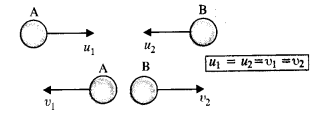Impulse = change in momentum
Initial momentum of each ball = 0.05 × 6 = 0.3 kg m/s
Final momentum of each ball = 0.05 × (-6) = -0.3 kg m/s
Impulse = 0.6 kgm/s (in magnitude).

Question 19.
A shell of mass 0.020 kg is fired by a gun of mass 100 kg. If the muzzle speed of the shell is 80 ms-1, what is the recoil speed of the gun?
Initially, gun and shell are at rest, thus Initial momentum = 0
Final momentum = momentum of bullet + momentum of gun = mbvb + mgvg By applying law of conservation of momentum,
Initial momentum of system = Final momentum of system
0 = mbvb + mgvg
mbvb =- mgvg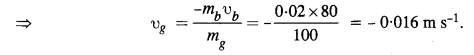Negative sign shows that gun moves backward as the shell moves forward.

Question 20.
A batsman deflects ability an angle of 45° without changing its initial speed which is equal to 54 km/h. What is the impulse imparted to the ball? (Mass of the ball is 0.15 kg.)
Suppose the point O is the position of bat. AO line shows the path along which the ball strikes the bat with velocity u and OB is the path showing deflection such that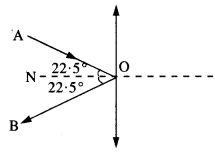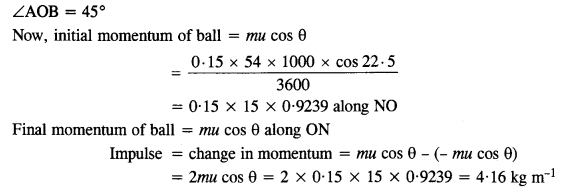Question 21.
A.stone of mass 0.25 kg tied to the end of a string is whirled round in a circle of radius 1.5 m with a speed of 40 rev./min in a horizontal plane. What is the tension in the string? What is the maximum speed with which the stone can be whirled around if the string can withstand a maximum tension of 200 N?
M = 0.25 kg, r = lm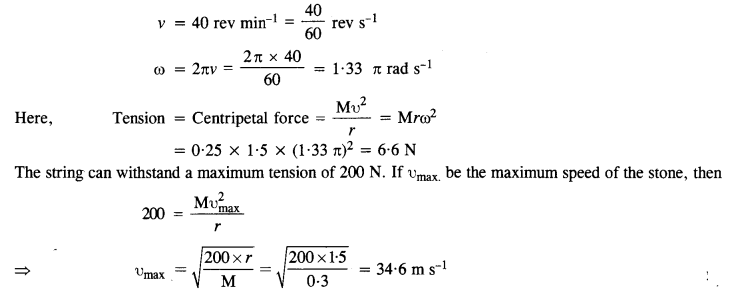Question 22.
If in Q. 21 the speed of the stone is increased beyond the maximum permissible value, and the string breaks suddenly, which of the following correctly describes the trajectory of the stone after the string breaks :
(a) the stone jerks radially outwards,
(b) the stoneflies oft tangentially from the instant the string breaks.
(c) the stoneflies oft at an angle with the tangent whose magnitude depends on the speed of the particle?
(b) Stoneflies off tangentially from the instant the string breaks due to inertia of direction.

Question 23.
For ordinary terrestrial experiments, which of the observers below are inertial and which non-inertial:

1. a child revolving in a “giant wheel”,
2. a driver in a sports car moving with a constant high speed of 200 km/h on a straight road,
3. the pilot of an aeroplane which is taking oft,
4. a cyclist negotiating a sharp turn,
5. The guard of a train which is slowing down to stop at a station?

1. In accordance with Newton’s 1st law of motion since there is no external agent the horse cannot pull cart.
2. The passenger continues to move forward when a speeding bus breaks because of their inertia of motion. Hence they are thrown forward from their seats.
3.  A lawn mover is pulled or pushed by applying a force at an angle. When it is pushed, the normal force (N) must be more than its weight, for equilibrium in the vertical direction. This results in greater friction and hence greater applied force to move. It is just opposite while pulling.
4. The ball will have a large momentum. If the player tries to stop it instantaneously, the time of contact is low which results in a large impulse which may hurt his hand. Hence he tries to move his hands backward which increases the time of contact hence reducing the impulse.

Question 24.
Explain why
(a) a horse cannot pull a cart and run in empty space,
(b) passengers are thrown forward from their seats when a speeding bus stops suddenly,
(c) it is easier to pull a lawnmower than to push it,
(d) a cricketer moves his hands backward while holding a catch.
(a) A horse on earth pushes the earth with its feet. According to Newton’s third law of motion, the earth exerts a reaction equal to push on the horse. Hence, horse moves forward and pulls the cart. No reaction is available in empty space and hence horse cannot pull the cart and run in empty space.
(b) Due to inertia of motion.
(c) During pull, the effective weight is reduced due to the vertical component of the pull. In the case of push, the vertical component increases the effective weight.
(d) By increasing time to stop the ball or decreasing its momentum to zero, force is reduced.

Question 25.
The figure shows the position-time graph of a particle of mass 0.04 kg. Suggest a suitable physical context for this motion. What is the time between two consecutive impulses received by the particle? What is the magnitude of each impulse?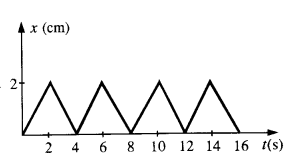This graph can be of a ball rebounding between two walls situated at positions 0 cm and 2 cm. The ball is rebounding from one wall to another, time and again every 2s with uniform speed.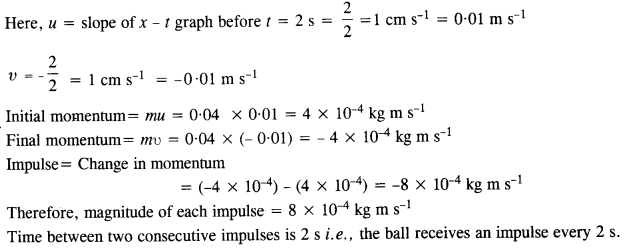Question 26.
Figure shows a man standing stationary with respect to a horizontal conveyor belt that is accelerating with 1 ms-2. What is the net force on the man ? If the coefficient of static friction between the man’s shoes and the belt is 0.2, up to what acceleration of the belt can the man continue to be stationary relative to the belt ? (Mass of the man = 65 kg.)Acceleration of belt, a = 1 ms-2, μs = 0.2
Net force on the man = mass of man x a = 65 x 1 = 65 N (y man is stationary w.r.t. belt)
The direction of this force is opposite to the direction of motion of the belt.
If a’ is the acceleration of the belt up to which the man can continue to be stationary relative to the belt, then
ma’ = maximum value of static friction
ma’ = μsR
ma’ =μmg or a’= μg
∴ d = 0.2 x 9.8 = 1.96 ms-2

Question 27.
A stone of mass m tied to the end of a string is revolved in a vertical circle of radius R. The net forces at the lowest and highest points of the circle directed vertically downwards are :
[Choose the correct alternative]Here T1, T2 ( υand υ2)) denote the tension in the string (and the speed of the stone) at the lowest and the highest point respectively.
(a)

Question 28.
A helicopter of mass 1000 kg rises with a vertical acceleration of 15 m s-2. The crew and the passengers weigh 300 kg. Give the magnitude and direction of the
(a) force on the floor by the crew and passengers,
(b) action of the rotor of the helicopter on the surrounding air,
(c) force op the helicopter due to the surrounding air.
(a) Force on the floor by the crew and passengers = apparent weight = m (a + g)
= 300 x (15 + 10) = 7500 N (vertically downwards)
(b) Action of the rotor of the helicopter on the surrounding air= apparent weight of helicopter, crew and passengers = (M + m) (a + g) = (1000 + 300) x (15 + 10) = 32500 N (vertically downwards)
(c) Applying Newton’s III rd law of motion, we find that the force on the helicopter due to the surrounding air is equal and opposite to the action of rotor on the surrounding air = 3500 N (vertically upward).

Question 29.
A steam of water flowing horizontally with a speed of 15 ms-1 gushes out of a tube of cross-sectional area 10-2 m2, and hits at a vertical wall near by. What is the force exerted on the wall by the impact of water, assuming it does not rebound ?
Volume of water striking the wall per second = υ x A = 15 x 102 = 0-15 m3s-1
Mass of water hitting wall per second, M = p x υ x A = 1000 x 0.15 = 150 kg s_1
Initial momentum of water per second = Mυ = 150 x 15 = 2250 kg m s1
Final momentum of water per second = 0    ( ∴ there is no rebound of water)
Magnitude of force = Rate of change of momentum = 2250 N.

Question 30.
Ten one-rupee coins are put on top of each other on a table. Each coin has a mass m kg. Give the magnitude and direction of
(a) the force on the 7th coin (counted from the bottom) due to all the coins on its top.
(b) the force on the 7th coin by the eighth coin,
(c) the reaction of the 6th coin on the 7th
(a) The seventh coin will experience force equal to the sum of weights of the three coins above it. As each count is of m kg, therefore
force on seventh coin = 3m kgf = 3 mg N
where   g = acceleration due to gravity.
(b) The eighth coin supports the weights of two coins above it. Therefore, the force on seventh coin due to the eighth coin will be equal to the sum of the weights of the eighth coin and the two coins above it.
Therefore, force on seventh coin due to eight coin = m + 2m = 3m kgf = 3mg N
(c) The sixth coin experiences force equal to weight of th.e four coins above it. Hence, reaction due to sixth coin on the seventh coin = 4 m kgf = 4 mgf.

Question 31.
An aircraft executes a horizontal loop at a speed of 720 km/h with its wings banked at 15°. What is the radius of the loop ?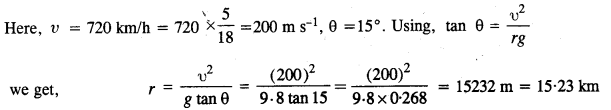Question 32.
A train rounds an unbanked circular bend of radius 30 m at a speed of 54 km/h. The mass of the train is 106 kg. What provides the centripetal force required for this purpose ? The engine or the rails ? What is the angle of banking required to prevent wearing out of the rail ?
(1) The centripetal force is provided by the lateral force acting due to outer rails on the wheels of the train.
(2) The outer rails will wear out faster as train exerts force (reaction) on them.
(3)Question 33.
A block of mass 25 kg is raised by a 50 kg man in two different ways as slown in figure. What is the action on the floor by the man in the two cases ? If the floor yields to a normal force of 700 N, which mode should the man adopt to lift the block without the floor yielding ?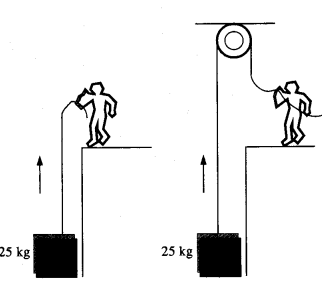In Ist case, man applies an upward force of 25 kg wt., (same as the weight of the block). According to Newton’s third law of motion, there will be a downward reaction on the floor.The action on the floor by the man
= 50 kg wt. + 25 kg wt.
= 75 kg wt = 75 x 9.8 = 735 N.
In case II, the man applies a downward force of 25 kg wt. According to Newton’s IIIrd law, the reaction is in the upward direction.
In this case, action on the floor by the man
= 50 kg wt. – 25 kg wt. = 25 kg wt = 25 X 9.8 = 245 N
∴ Man should adopt the second method.

Question 34.
A monkey of mass 40 kg climbs on a rope (Fig.) which can stand a maximum tension of 600 N. In which of the following cases will the rope break : the monkey
(a) climbs up with an acceleration of 6 ms2
(b) climbs down with an acceleration of 4 ms2
(c) climbs up with a uniform speed of 5 ms1
(d) falls down the rope nearly freely under gravity ?
(Ignore the mass of the rope.)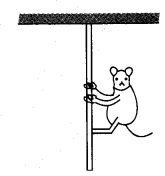Maximum tension the rope can stand = 600 N, g = 10 ms-2
Mass of monkey = M = 40 kg
(a) Here a = 6 ms2 (upwards)
∴ apparent weight of the monkey = M (g + a) = 40 (10 + 6) = 640 N
Since the maximum permissible tension is 600 N, thus the rope will break.

(b)
Here a 4ms2(downwards)
∴ apparent weight of the monkey = tension in the rope
= M(g – a) = 40 (10 – 4) = 240 N
Thus rope will not break.

(c)
Here uniform velocity = 5ms, so a = 0
∴ tension in the rope = M(g + a) = 40(10 + 0) = 400 N
Thus the rope will not break.

(d)
When the monkey falls down freely under gravity, it is in the state of weightlessness. As there will be no tension in the string, the rope will not break.

Question 35.
Two bodies A and B of masses 5 kg and 10 kg in contact with each other rest on a
table against a rigid partition (Fig.). The co-efficient of friction between the bodies
and the table is 015. A force of 200 N is applied horizontally at A. What are
(a) the reaction of the partition (b) the action-reaction forces between A and B ? What happens when the partition is removed ? Does the answer to (b) change, when the
bodies are in motion ? Ignore the difference between μs and μk
(a) Limiting force of friction, F1 = μR= μ (mA+ mB) g = O.15(5 + 10) 9.8 = 22.05 N (towards left)
Net force on the partition = F – Ff = 200 — 22.05 = 177.95 N
:. Reaction of partition = -177.95 N
(b) Force of friction on A, FA = μmAg = 0.15 x 5x 9.8 = 7.35 N
;. Net force exerted by A on B
=(F-FA) =200-7.35=19265 NQuestion 36.
A block of mass 15 kg is placed on a long trolley. The co-efficient of static friction between the block and the trolley is 0.18. The trolley accelerates from rest with 0.5 ms-2 for 20 s and then moves with uniform velocity. Discuss the motion of the block as viewed by
(a) a stationary observer on the ground,
(b) an observer moving with the trolley.
(a) Force experienced by block, F = ma = 15 x 0.5 = 7.5 N
Force of friction, Ff – μmg = 0.18 x 15 x 9.8 = 26.46 N
i.e..force experienced by block is less than the friction, so the block will not move. It will remain stationary w.r.t. trolley for a stationary observer on ground.

(b) The observer moving with trolley has an accelerated motion i.e. he forms non inertial frame in which Newton’s laws of motion are not applicable. The box will be at rest relative to the observer.

Question 37.
The rear side of a truck is open and a box of 40 kg mass is placed 5 m away from the open end as shown in Fig.The co-efficient of friction between the box and the surface below it is 0.15. On a straight road, the truck starts from rest and accelerates with 2 ms-2. At what distance from the starting point does the box fall off the truck ? (Ignore the size of the box).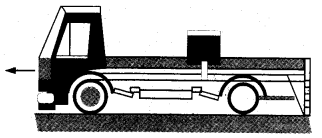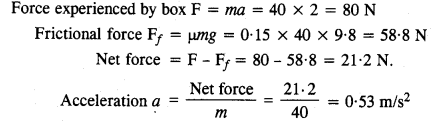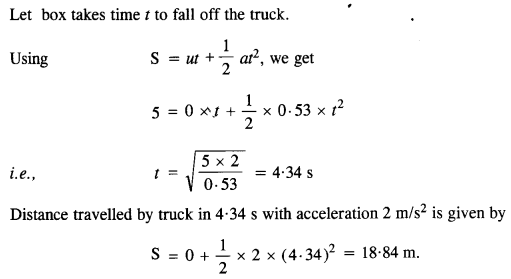Question 38.
A disc revolves with a speed of 33$$\frac { 1}{ 3 }$$  rev/min, and has a radius of 15 cm. Two coins are placed 4 cm and 14 cm away from the center of the record. If the co-efficient of friction between the coins and the record is 0.15, which of the coins will revolve with the record ?
The coin can only revolve with the record when the force of friction is enough to provide the centripetal force. If this force is riot enough then the coin slips on the record.
Here  R = mg and the centripetal force is given by mrω2
Therefore, to prevent slipping, the condition should be μmg ≥ mm
or μg ≥ rω2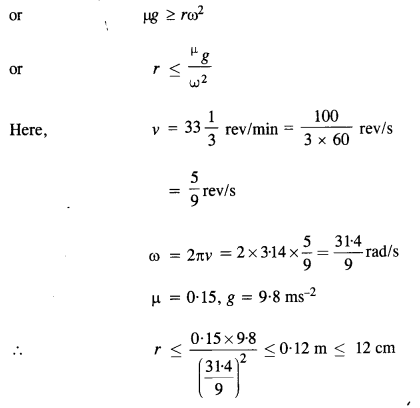Thus, coin placed at 4 cm continues to rotate with the record.

Question 39.
You may have seen in a circus a motorcyclist driving in vertical loops inside a ‘death-well’ (a hollow spherical chamber with holes, so the spectators can watch from outside). Explain clearly why the motorcyclist does not drop down when he is at the uppermost point, with no support from below. What is the minimum speed required at the uppermost position to perform a vertical loop if the radius of the chamber is 25 m ?
At the point top of the loop, the equation of motion is given by(1)
Where R is Normal reaction and r is radius of loop. Minimum possible speed at uppermost point is when R = 0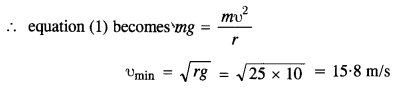Question 40.
A 70 kg man stands in contact against the inner wall of a hollow cylindrical drum of radius 3 m rotating about its vertical axis with 200 rev/min. The coefficient of friction between the wall and his clothing is
0.15. What is the minimum rotational speed of the cylinder to enable the man to remain stuck of the wall (without falling) when the floor is suddenly removed?
The horizontal reaction R of the wall on the man provides the necessary centripetal force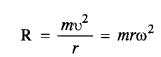The frictional force/acting upwards balances the weight mg of the man.
The man will remain stuck to the wall after the floor is removedQuestion 41.
A thin circular wire of radius R rotates about its vertical diameter with an angular frequency co. Show that a small bead on the wire remains at its lowermost point for ω≤ $$\sqrt { g/r }$$. What is the angle made by the radius vector joining the center to the bead with the vertical downward direction for ω= $$\sqrt { 2g/r }$$? Neglect friction.